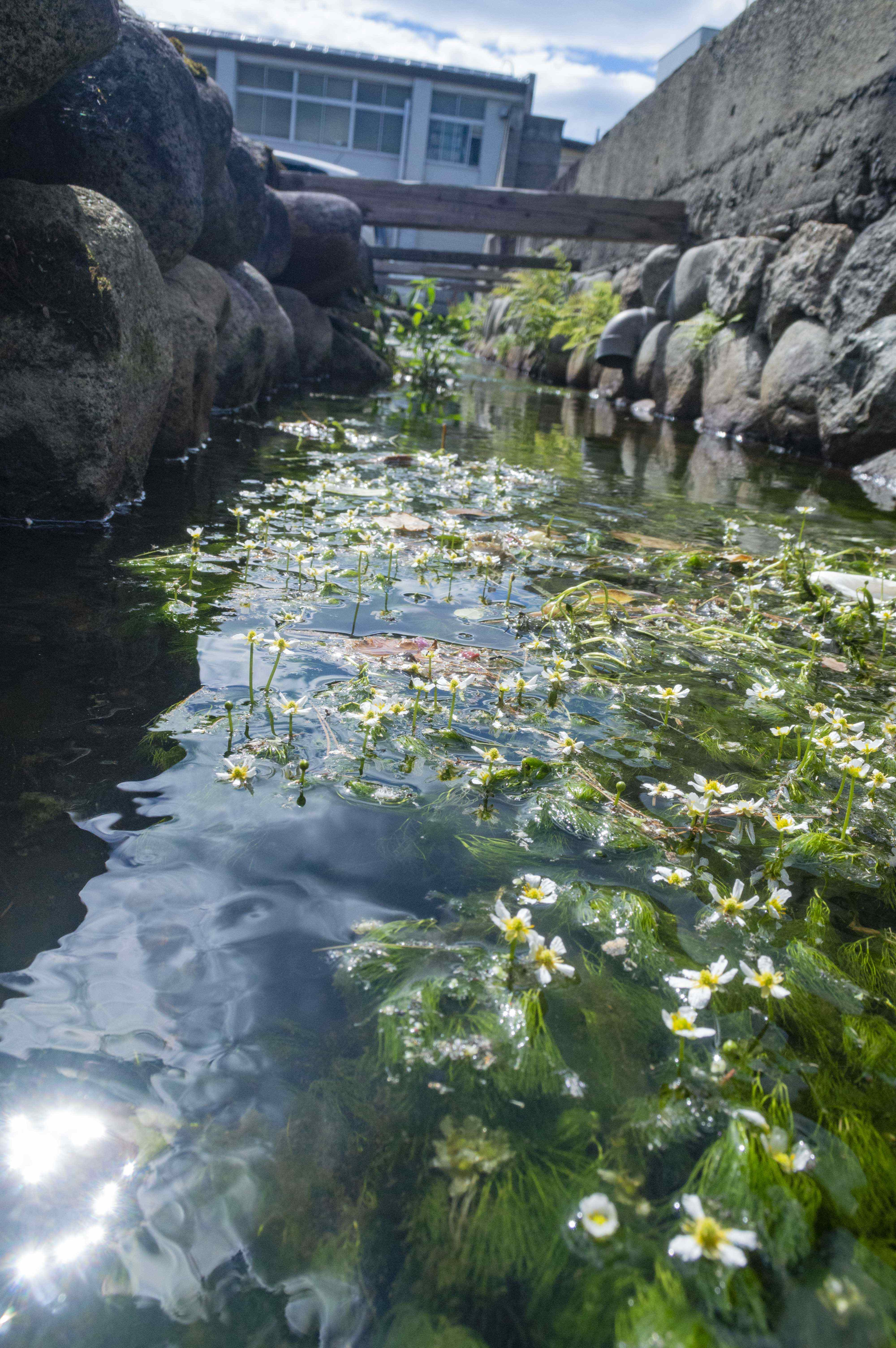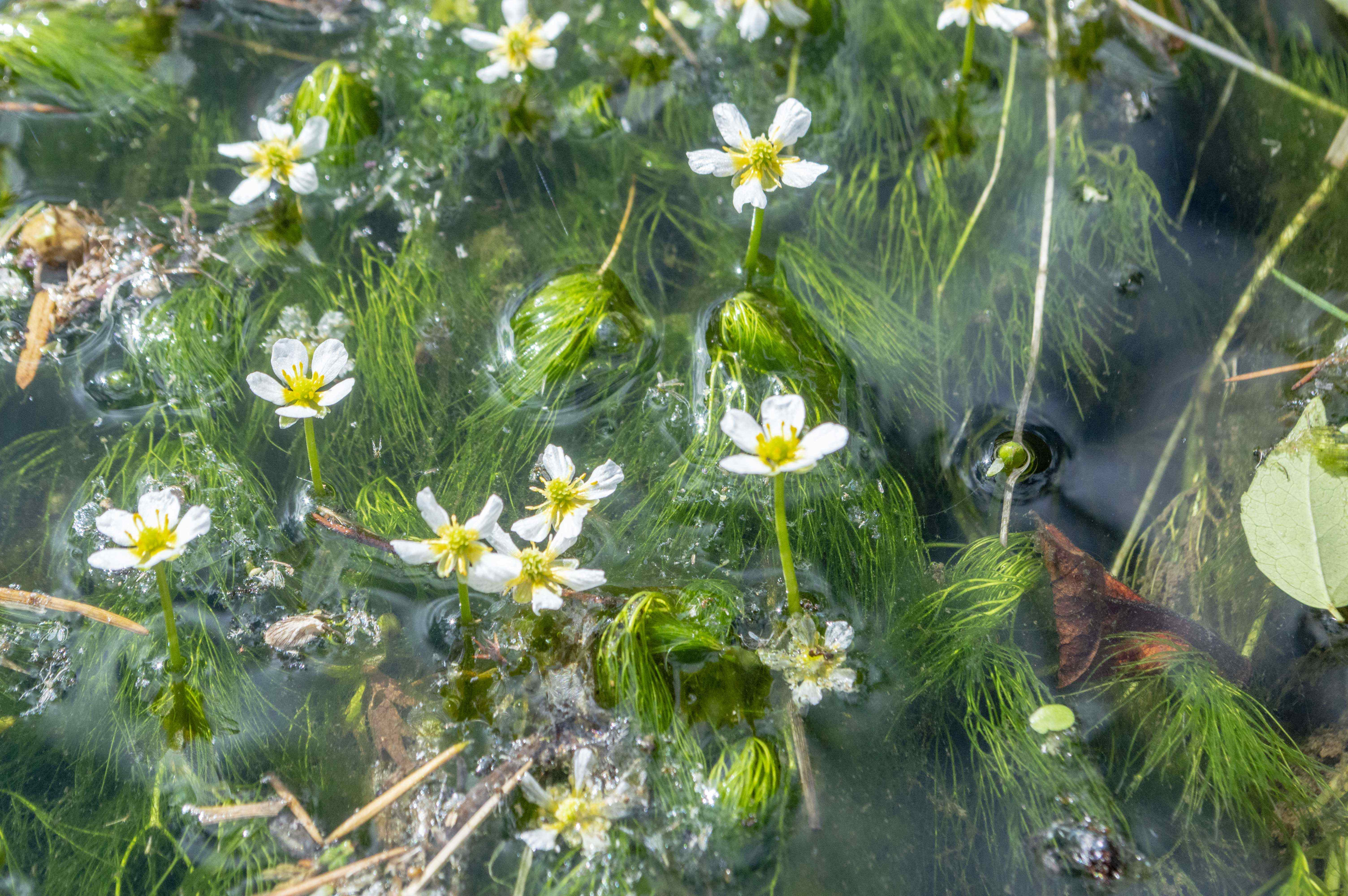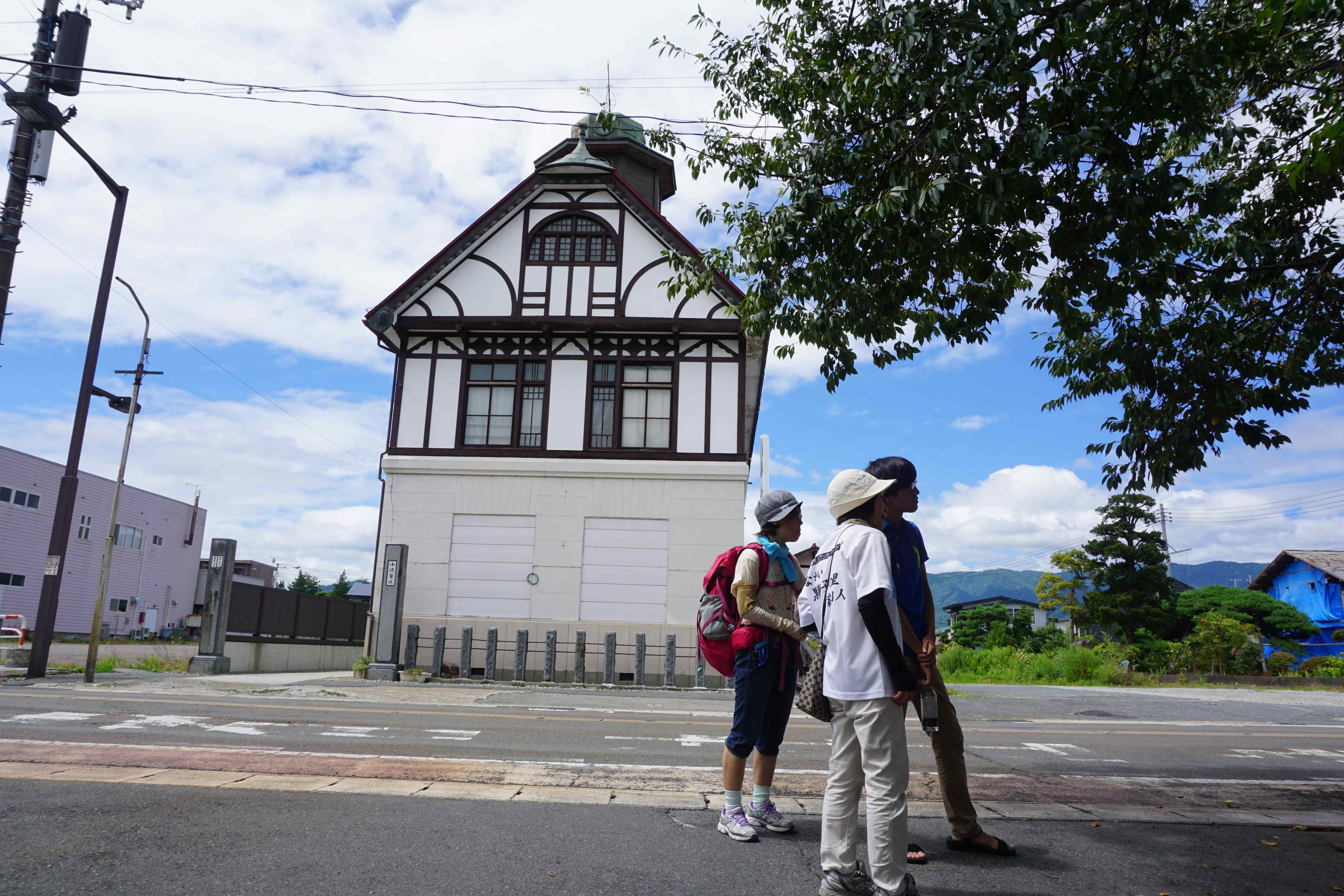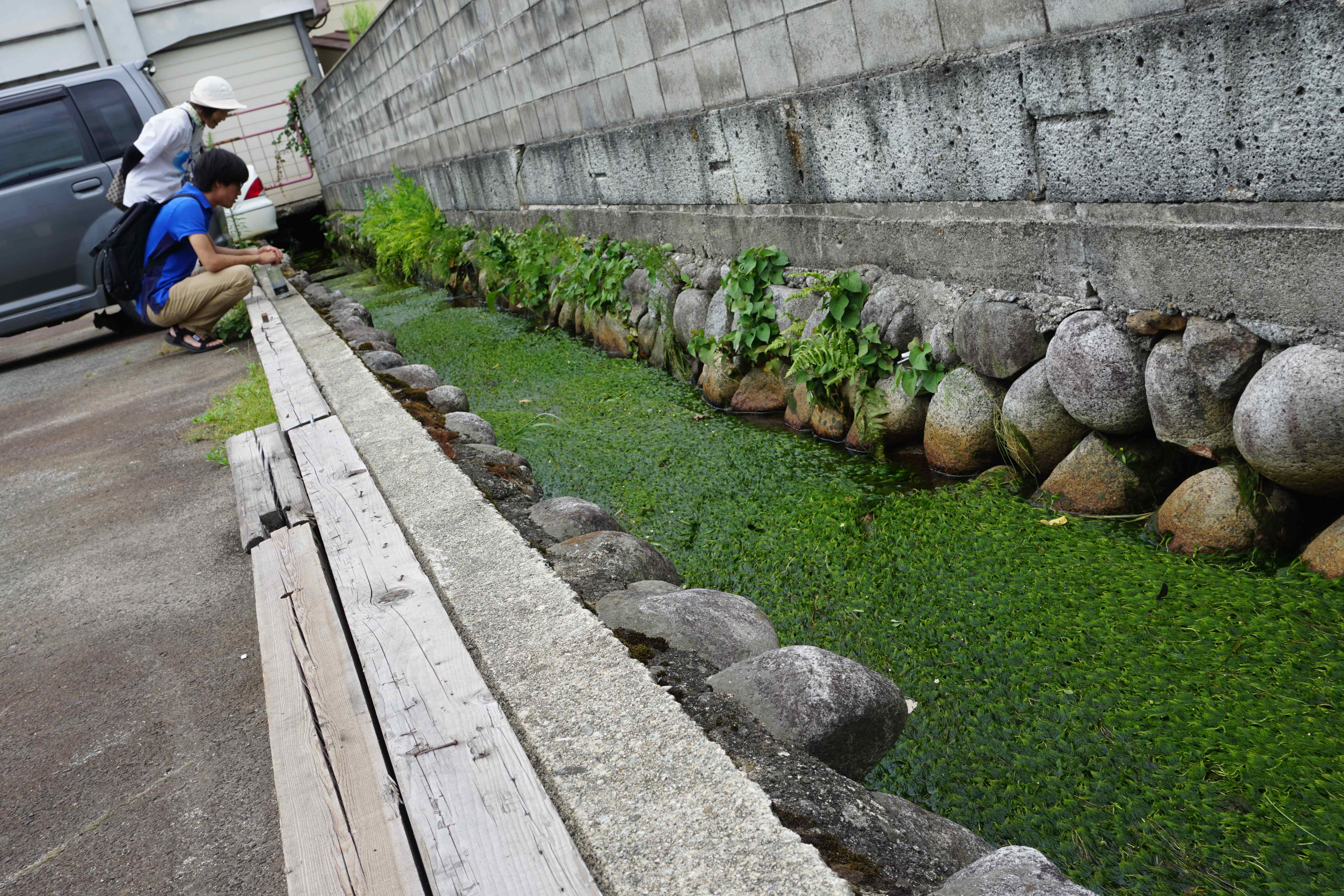# 文章詳細頁

## [梅花藻]8月10日開花信息※為了請在參觀之際在梅花藻看護車的停車禮儀請求合作。

=+=+=+=+=+=+=+=+=+=+=+=+=+=+=+=+=+=+=+=+=+=+=+=+=2018.08.10:[市町村新聞]

## 對這篇文章的評語是這裡

• 名字
• 題名
• 正文
• URL
• ▼供編輯/刪掉使用的密碼(請在半角英文和數字4個字自由地輸入)
• ▼手動輸入確認(請用半角英國文字/小寫字母輸入圖片的文字)• 　※任何人投稿可的/立刻，并且反映

### 山形長井觀光局|秘書處vol.2108,892件合計170,440,968件今天18,635件昨天